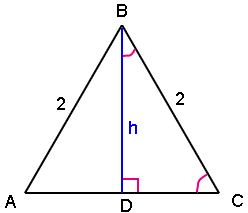### Trigonometry: Finding the value of sine Pi/3.

Trigonometry: Finding the value of sine Pi/3.

First we need to remember that the whole circle is 360° and in radians it is 2Pi. So then Pi is 180°, and Pi/3 is 60°.

To find sine of Pi/3, you'd want to have a right triangle with one angle 60°.

Fortunately that is easy to come by; just take an equilateral triangle and draw an altitude to it. You will have two identical 30°-60°-90° triangles.

And yes this is one of the special triangles - also used in drafting, and there are rulers in this shape.Where on this picture is the 60° angle? Where's the 30° angle?

Now, to get sine 60° one needs side lengths. I made the sides of this equilateral triangle ABC to be 2 units. The side CD is obviously just 1 unit (easy numbers thus far!)

But what about the height h?

Well, that's where we need to dig up the goold ole' Pythagoras. Can't forget him.

You write the equation, h2 + 12 = 22

h2 = 22 − 12 = 3.

So taking square roots... h = √3.

Then, to the sine.

Remember sine is a ratio of side lengths; it is the ratio of the OPPOSITE side to the hypotenuse.

....and soon you will have the answer: sine 60° is _____ (fill in the blank.)

So it was easy, just using the very basics of trigonometry.

However, I wouldn't memorize the result. Just remember the idea HOW it was derived; and you can derive it when you need it (such as in a test).

Special Right Triangles